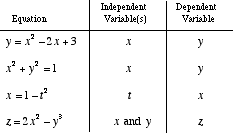dependent variable

Dependent Variable

A variable that depends on one or more other variables. For equations such as y = 3x – 2, the dependent variable is y. The value of y depends on the value chosen for x. Usually the dependent variable is isolated on one side of an equation. Formally, a dependent variable is a variable in an expression, equation, or function that has its value determined by the choice of value(s) of other variable(s).